##### The Japanese Bonsai specialist
Direct order Contact Help / Services Newsletter# Pinus pentaphylla ref: 19090223

Pinus pentaphylla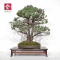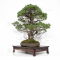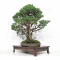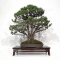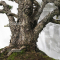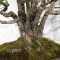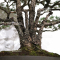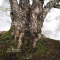ref. : 11781

2.650,00

voluminous/heavy item extra shipping of 12,00

Available quantity : 1Order

###### Description

This tree is a mix of multi-trunks and a lettered tree, it's a unique piece. A lot of emotion with the softness of its trunks. It comes from Master Shiino Kentaro's nursery.

Maximum height of the tree: 790 mm. Width of the branches: 770 mm. Japanese old quality artisanal firmed non-enameled pot: 450*330**60 mm.

Little needles that are naturally short and thin. Kabudachi style with 7 trunks. This bonsaï is more than 70 years old with its perfectly formed bark, coming from yamadori more than 40 years ago.

Well-ramified and good conicity of the trunks.

No grafting mark as it is a natural seedling, nor strong wire or cutting wound.

Dimensions of the trunks: 20-25-35 mm and 110 mm at the base of the 7 trunks.

Dimensions of the superb and regular nebari: 220*300 mm. Quality subject for a collector who will take time to cut it regularly in order to densify its trays. Admire the natural beauty of each one of its trunks and the grace of their curves.

Photographed in September. Shelf not included. Reformed by master Tomoya Nishikawa in September 2018.

#trunks 7.6 #bonsai 3.6 #tree 3.4 #with 3.4 #pentaphylla 3.1 #dimensions 3 #september 2.9 #quality 2.7 #natural 2.7 #outdoor 2.7

Formule
(( ROUND((CHAR_LENGTH(b.article_nom)-CHAR_LENGTH(REPLACE(b.article_nom, 'trunks', '')))/LENGTH('trunks')) + ROUND((CHAR_LENGTH(b.article_description)-CHAR_LENGTH(REPLACE(b.article_description, 'trunks', '')))/LENGTH('trunks')) ) * 7.6) + (( ROUND((CHAR_LENGTH(b.article_nom)-CHAR_LENGTH(REPLACE(b.article_nom, 'with', '')))/LENGTH('with')) + ROUND((CHAR_LENGTH(b.article_description)-CHAR_LENGTH(REPLACE(b.article_description, 'with', '')))/LENGTH('with')) ) * 3.4) + (( ROUND((CHAR_LENGTH(b.article_nom)-CHAR_LENGTH(REPLACE(b.article_nom, 'tree', '')))/LENGTH('tree')) + ROUND((CHAR_LENGTH(b.article_description)-CHAR_LENGTH(REPLACE(b.article_description, 'tree', '')))/LENGTH('tree')) ) * 3.4) + (( ROUND((CHAR_LENGTH(b.article_nom)-CHAR_LENGTH(REPLACE(b.article_nom, 'pentaphylla', '')))/LENGTH('pentaphylla')) + ROUND((CHAR_LENGTH(b.article_description)-CHAR_LENGTH(REPLACE(b.article_description, 'pentaphylla', '')))/LENGTH('pentaphylla')) ) * 3.1) + (( ROUND((CHAR_LENGTH(b.article_nom)-CHAR_LENGTH(REPLACE(b.article_nom, 'dimensions', '')))/LENGTH('dimensions')) + ROUND((CHAR_LENGTH(b.article_description)-CHAR_LENGTH(REPLACE(b.article_description, 'dimensions', '')))/LENGTH('dimensions')) ) * 3) + (( ROUND((CHAR_LENGTH(b.article_nom)-CHAR_LENGTH(REPLACE(b.article_nom, 'september', '')))/LENGTH('september')) + ROUND((CHAR_LENGTH(b.article_description)-CHAR_LENGTH(REPLACE(b.article_description, 'september', '')))/LENGTH('september')) ) * 2.9) + (( ROUND((CHAR_LENGTH(b.article_nom)-CHAR_LENGTH(REPLACE(b.article_nom, 'quality', '')))/LENGTH('quality')) + ROUND((CHAR_LENGTH(b.article_description)-CHAR_LENGTH(REPLACE(b.article_description, 'quality', '')))/LENGTH('quality')) ) * 2.7) + (( ROUND((CHAR_LENGTH(b.article_nom)-CHAR_LENGTH(REPLACE(b.article_nom, 'natural', '')))/LENGTH('natural')) + ROUND((CHAR_LENGTH(b.article_description)-CHAR_LENGTH(REPLACE(b.article_description, 'natural', '')))/LENGTH('natural')) ) * 2.7) + (( ROUND((CHAR_LENGTH(b.article_nom)-CHAR_LENGTH(REPLACE(b.article_nom, 'master', '')))/LENGTH('master')) + ROUND((CHAR_LENGTH(b.article_description)-CHAR_LENGTH(REPLACE(b.article_description, 'master', '')))/LENGTH('master')) ) * 2.6) + (( ROUND((CHAR_LENGTH(b.article_nom)-CHAR_LENGTH(REPLACE(b.article_nom, 'pinus', '')))/LENGTH('pinus')) + ROUND((CHAR_LENGTH(b.article_description)-CHAR_LENGTH(REPLACE(b.article_description, 'pinus', '')))/LENGTH('pinus')) ) * 2.5)

## Secure payment## Delivery

Our logistic partners :04 74 55 23 48
Pépinière MAILLOT-BONSAÏ
Le Bois Frazy
01990 RELEVANT - FRANCE
on appointment